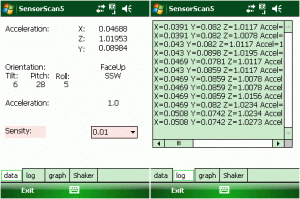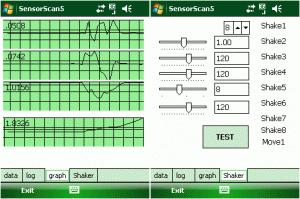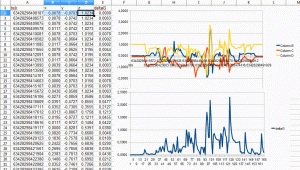## Mobile Development – Shake that thing

it has been a long time since my last post, I was a little bit busy.

This time I want to present some experimental code to visualize and analyze G-Sensor data. The goal was to achieve a shake detection algorithm. Unfortunately the device under test only provided 1 sample per second and that is not enough for a good shake detection. Beside that the code and classes developed may help you to find your way and they help you at last to determine the current orientation of the device.

left shows general information taken from vector, right shows a log with last vector data[image SensorScan5_0102.gif][image SensorScan5_0304.gif]
left shows graphical of vector and force (length), right shows indicators for detected events

A g-sensor or accelerometer sensor normally gives you the x, y and z-values of a vector. A vector is an imaginary arrow with a direction and length starting from the three dimensional point 0,0,0. The vector direction points to the acceleration of the device. The normal acceleration on earth is 9,81m/s^2. If the device is on the desk, the y-acceleration is about minus 9.81m/s^2. The absolute value of the sensor may vary on the sensor and maybe defined as 1.0 for -9.81m/s^2 or -0.981. If you through the device up to the air, the x,y and z-values will reach 0,0,0 as if the device is weightless. Keep in mind that the acceleration towards the middle of the earth is always there and the device will come back to you.

The device is facing upwards (see y arrow) with the top facing to you (the z arrow). The left side of the device is pointing to the right (the x arrow).

The light green/blue and the yellow arrows demonstrate two different vectors which show the direction (the xyz angles) and the force (the vector lengths) to the device.

and here is an image about how the vector and the device is aligned:

```#region CN50 XYZ vectors
/*
(N)
+Y
|           -Z
|          /
|         /
|       .'
+-----------+/
| +-------+ |
| |       | |
| |       | |
| |       | |
(E)''''''| |  /    | |''''''  (W)
+X       | |.'     | |     -X
| /-------+ |
|/          |
.'           |
/ +-----------+
.'        |
/          |
+Z           |
-Y
(S)

//calc the acceleration or the longest vector
double accel = Math.Sqrt(x * x + y * y + z * z);
//calc the X angle, should be about 180 if device is upright
double degrees = Math.Acos(x / b) * 360.0 / Math.PI;

* when device is faceup on table:          x=0     y=0     z=1
* when device is faceup on table:          x=0     y=0     z=-1
* when device is upright scan to roof:     x=0     y=-1    z=0
* when device is upright scanner to down:  x=0     y=1     z=0
* when scanner lays on left side:          x=-1    y=0     z=0
* when scanner lays on right side:         x=1     y=0     z=0
*/
```

The development device was an Int*rm*c CN50. To be able to get the sensor data into a .NET app, first install the sensor wrapper DLLs (sensor.cab) of the Int*rm*c Device Resource Kit. For other devices you just have to change the code that is used to register for sensor data changes.

```		mySensor.AccelerationEvent += new AccelerationEventHandler(mySensor_AccelerationEvent);
...
void mySensor_AccelerationEvent(object sender, Sensor.AccelerationArgs AccelerationArgs)
{
ShakeDetection.GVector gv = new ShakeDetection.GVector(AccelerationArgs.GForceX,
AccelerationArgs.GForceY,
AccelerationArgs.GForceZ);

processData(gv);
}
```

The class GVector implements some analysis on the vector data. The vector data is built of three acceleration values, the X, Y, and Z part of the acceleration. If the device is layed on a desk, the Z vector is about -9.81 m/s and the X and Y vector will be at 0.0 m/s. If you move the device or turn it, the xyz values will change and you can calculate an orientation and if you collect and evaluate the data over time, you can get the acceleration of the device. If it is falling, the xyz values will all be zero.

The processData() call updates the GUI and feeds the vector data to the shake and movement detection classes:

```        private void processData(ShakeDetection.GVector gv)
{
if (starting)
return;
labelGFX.Text = gv.X.ToString("0.00000");
labelGFY.Text = gv.Y.ToString("0.00000");
labelGFZ.Text = gv.Z.ToString("0.00000");

lblOrientation.Text = gv.ToScreenOrientation().ToString();
lblDirection.Text = gv.direction.ToString();

lblTilt.Text = gv.Tilt.ToString("0");
lblRoll.Text = gv.Roll.ToString("0");
lblPitch.Text = gv.Pitch.ToString("0");

lblAcceleration.Text = gv.Length.ToString("0.0");

...
```

The movement classes use an extended version of the GVector class called GMVector. The movement classes are implemented with the basic GMVector interface:

```namespace Movedetection
{
public struct GMVector
{
public GMVector(double x, double y, double z)
{
myX = x;
myY = y;
myZ = z;
myTicks = (ulong)(DateTime.Now.Ticks / 10000); //there are 10000 ticks in a millisecond
// http://msdn.microsoft.com/en-us/library/system.datetime.ticks.aspx

myDirection = GetDirection(myX, myY, myZ);
myScreenOrientation = GetScreenorientation(myX, myY, myZ);
moveState = MoveState.idle;
}
...
```

The idea was to implement a movement analysis and compare the movement of the device with a given pattern. For example if the last movement sequence matches the given pattern UP-LEFT-DOWN an event will be generated. BUT this is not yet implemented!

The shake and movement classes are all derived from a base class that defines a common interface:

```using System;

namespace ShakeDetection
{
public interface IShake
{
void OnShakeDetected(GVector gv);
}

}
```

Inside the derived classes the vector data is processed and the shake or movement ‘calculation’ is implemented. All shake classes are derived from a basic class called “ShakeClass” (or “Movement” class):

```namespace ShakeDetection
{
///

/// Main abstract class to implement various Shake detector classes
///

public abstract class ShakeClass:IShake,IDisposable
{
#region Properties
private string _name = "ShakeClass";
///

/// used to identify multiple classes that inherited this class
///

public string name{
get{return _name;}
set { _name = value; }
}
...
```

One analysis done by GVector class itself is the segmented direction, which shows in which direction the top of the device is pointing expressed in compass like directions.

```		#region segmented direction
// http://en.wikipedia.org/wiki/Boxing_the_compass" href="http://en.wikipedia.org/wiki/Boxing_the_compass"
//attention: this here is based on +Y/+X (-1/0) equal 0 degree equal North
public enum Direction:int{
None=-1,
W=0,
WSW,
SW,
SSW,
S,
SSE,
SE,
ESE,
E,
ENE,
NE,
NNE,
N,
NNW,
NW,
WNW,
}
public Direction direction
{ // http://stackoverflow.com/questions/1437790/how-to-snap-a-directional-2d-vector-to-a-compass-n-ne-e-se-s-sw-w-nw
get{
GVector gv=this;
int segmentCount=16;
int compassSegment = (((int) Math.Round(Math.Atan2(gv.Y, gv.X) / (2 * Math.PI / segmentCount))) + segmentCount) % segmentCount;
return (Direction)compassSegment;
}
}
#endregion
```

Another simply analysis gives you the orientation of the device, that means where the display is facing to.

```            //changed to match CN50 XYZ directions
public ScreenOrientation ToScreenOrientation()
{
if (Math.Abs(X) > Math.Abs(Y))
{
if (Math.Abs(X) > Math.Abs(Z))
{
if (X > 0)
return ScreenOrientation.ReverseLandscape;     //changed from Landscape
return ScreenOrientation.Landscape;                //changed from ReverseLandscape
}
}
else if (Math.Abs(Y) > Math.Abs(Z))
{
if (Y > 0)
return ScreenOrientation.ReversePortrait;       //changed from Portrait
return ScreenOrientation.Portrait;                  // changed from ReversePortrait
}

if (Z > 0)
return ScreenOrientation.FaceUp;    //this is different to HTC
return ScreenOrientation.FaceDown;      //this is different to HTC
}
}
```

When you take a look at the vectors when you move the device slow and fast, you will see the xyz values changing and if you add the absolute length over the time, you will get a cumulated ‘force’.[image vectors-analysis.gif]
the upper graphics shows the x,y,z values of the sensor and the lower graphic shows the accumulated force over the time

The shaker classes implement different ‘shake’ detection algorithms. The simplest one is to add current force value to a cache and compare the average values with a defined threshold, see ShakeClass1.cs:

```...
///
/// this function uses every direction for its own shake detection
///

//code is from android where 9.8m/s^² is normal
//cn50 gives 0.98m/s^² so we multiply the CN50 values with 10
gv = gv.Scale(10);

this.Logger(gv.ToString());
if(isShaking(_X_Cache, gv.X) ||
isShaking(_Y_Cache, gv.Y) ||
isShaking(_Z_Cache, gv.Z) )
{
this.OnShakeDetected(gv);
}
}
...
private bool isShaking(Queue cache, double currentValue)
{
double average = 0;
foreach (double d in cache)
{
average += d;
}
average = average / cache.Count;
this.Logger(String.Format("ShakeClass1: isShaking: average: {0} current: {1} treshold:{2} diff:{3}", average, currentValue, _shakeTreshold, Math.Abs(average - currentValue)));
return Math.Abs(average - Math.Abs(currentValue)) > _shakeTreshold;
}
...
```

```using System;
using System.Collections.Generic;
using System.Text;

namespace Movedetection
{
/// challenge: recognize a gesture pattern
/// Detect movement (Accelerometer_WBSN.pdf)
/// 1. calc the actual acceleration: Length=sqrt(x*x+y*y+z*z)
/// 2. get the diff to the previous DeltaAcceleration: LengthNow - LengthPrevious
/// 3. get the average of deltas for about 1 sec (should be 20 measurements): SUM(DeltaAccelerations)/sps; //sps=samples per second
///

class MovementClass1:MovementClass
{

public MovementClass1(string s)
{
base.name = s;
this.setTreshold(.2d, 0.5d);
myQueue = new LimitedQueue(_queueLength);

myAverages = new LimitedQueue(_queueLength);

//need at least 3 seconds recording size, but only record crossing values
myCrossUPs = new LimitedQueue(_queueLength);
myCrossDOWNs = new LimitedQueue(_queueLength);

myGmin = 0.2d; //was 0.9d but we get a deltaAverage of about around 0.14
myTmin = 10; // 15 is equal to 0.75 seconds if samples per seconds is 20

_treshCountRMS = 2000;  //should be 3000 for 60 samples in 3 seconds at 20 samples/second,
//BUT we only see ~2 samples per second

basicLogger("Movement1 Class\r\nx\ty\tz\ttick\tdeltaG");
}

public override void setTreshold(double high, double low)
{
myGmin = high;
myRMSmin = low;
}

{
GMVector gvOld;
if (myQueue.Count>0)// !_firstCall)
{
//_samplesPerSecond=
//calc samples per second
if (!_samplesPerSecondsCalulated)
{
if (myQueue.Count > 10)
{
GMVector[] myQueueArray = myQueue.ToArray();
ulong iSamplesCount = 0;
ulong iTickSum = 0;
ulong tickDiff = 0;
for (int c = myQueueArray.Length - 1; c > 1; c--)
{
iSamplesCount++;
tickDiff = myQueueArray[c].Ticks - myQueueArray[c - 1].Ticks;
iTickSum += tickDiff;
}
_samplesPerSecond = (uint)(1000 / (iTickSum / iSamplesCount)); // number of samples per second, ticks stored as milliseconds
_samplesPerSecondsCalulated = true;
}
}
//get and store delta
gvOld = myQueue.Peek();
//calculate deltaG for current and last sample
double deltaG = Math.Abs(gv.Length-gvOld.Length);

//save for later use
gRMSAverage currentRMS=new gRMSAverage(deltaG, gv.Ticks);
myAverages.Enqueue(currentRMS);

basicLogger(string.Format("{0}\t{1}\t{2}\t{3}\t{4}",
gv.X, gv.Y, gv.Z,
gv.Ticks,
deltaG));

/* Theory:
* RSD: Rapid Shake Detection
* acceleration change is the sum of the delta G between consecutive samples divided
* by the number of samples per second
* Drastic Movement
*  average acceleration change exceeds about 0.9G per sample (50ms period)
*  calculated over the last .75 seconds
* Sustained Movement
*  more fequently exceed an acceleration of about 0.5G (0.5G acceleration change per sample)
*  exceed 0.5G value within time frame for non-consecutive samples (cross counter)
*  time frame is set to reset after 60 non-consecutive (about 3 seconds at 50ms per sample) samples below 0.5G
*/

//test for condition1
bool bCondition1=false;
if (myAverages.Count > 1)
{
bCondition1 = condition1(currentRMS);
}

//test for condition2
bool bCondition2=false;
if (myAverages.Count > 1)
{
gRMSAverage lastRMS = myAverages.Peek();
bCondition2 = condition2(currentRMS, lastRMS);
}

//===============================================================================================
//start when queue is filled
if (myQueue.Count >= 20)
{
//rapid shake detection (RSD)
//Condition1: have at least an average of 0.9G for at least .75 second
if(bCondition1 & bCondition2)
OnMoveDetected(gv);
}
//else
//    _firstCall = false;

myQueue.Enqueue(gv);

gvOld = gv;
}

/* Theory:
* RSD: Rapid Shake Detection
* acceleration change is the sum of the delta G between consecutive samples divided
* by the number of samples per second
* Drastic Movement (condition1)
*  average acceleration change exceeds about 0.9G per sample (50ms period)
*  calculated over the last .75 seconds
*/
/// deltaG above 0.9G for at least .75 seconds
///
private bool condition1(gRMSAverage currentRMS)
{
if (!_samplesPerSecondsCalulated)
return false;

bool bRet1 = false;
//get average of values of last .75 seconds, tick diff is about 10ms between samples
double sum = 0;
int iSamples = 0;

foreach (gRMSAverage gRMS in myAverages)
{
if (currentRMS.tick - gRMS.tick < 75 * 1000) //the ticks saved are milliseconds, .75seconds=75000 milliseconds
{
sum += gRMS.deltaG;
iSamples++;
}
}
//sum of deltas divided by samples per second
double DeltaAverage = sum / _samplesPerSecond;// (iSamples * 4 / 3);

Avg_deltaG_rms = DeltaAverage;

//call back for information, the caller reads _Avg_deltaG_rms
OnIdleDetected(new GMVector(0, 0, 0));

//how many samples exceed the Gmin treshold
int iCountG = 0;
foreach (GMVector g in myQueue)
{
if (g.Length > DeltaAverage)
iCountG++;
}
//Condition1: have at least an average of 0.9G for at least .75 second
if (DeltaAverage > myGmin){
if (iCountG > myTmin)
{
//delete all entries, RESET
myQueue.Clear();
//_firstCall = true;

//OnMoveDetected(gv);
bRet1 = true;
}
}
return bRet1;
}

/// deltaG above .5G for more than 3 seconds and
/// without deltaG below .5G within last 3 seconds
private bool condition2(gRMSAverage currentRMS, gRMSAverage lastRMS){

if (!_samplesPerSecondsCalulated)
return false;

bool bRet = false;
crossDirection crossDir = crossDirection.unknown;
if (currentRMS.deltaG > myRMSmin && lastRMS.deltaG < myRMSmin)
{
//crossUp
crossDir = crossDirection.crossUP;
}
else if (currentRMS.deltaG < myRMSmin && lastRMS.deltaG > myRMSmin)
{
//crossDown
crossDir = crossDirection.crossDown;
}

if (myCrossDOWNs.Count > 0)
{
gRMSAverage lastRMSup = myCrossDOWNs.Peek();
if (crossDir == crossDirection.crossUP && (currentRMS.tick - lastRMSup.tick) >= _treshCountRMS)
{
if (myCrossUPs.Count > 0)
{
gRMSAverage lastRMSdown = myCrossDOWNs.Peek();
if ((currentRMS.tick - lastRMSdown.tick) > _treshCountRMS)
bRet = true;
}
}
}
//enqueue current values
if (crossDir == crossDirection.crossUP)
myCrossUPs.Enqueue(currentRMS);
if (crossDir == crossDirection.crossDown)
myCrossDOWNs.Enqueue(currentRMS);
return bRet;
}
#region fields
private uint _samplesPerSecond = 20;
private bool _samplesPerSecondsCalulated = false;

private enum crossDirection : int
{
unknown = 0,
crossUP = 1,
crossDown = -1,
}

private class gRMSAverage
{
private double _deltaG;
public double deltaG
{
get { return _deltaG; }
}
private ulong _tick;
public ulong tick
{
get { return _tick; }
}
public gRMSAverage(double delta, ulong tick)
{
_deltaG = delta;
_tick = tick;
}
}

/// store a list of acceleration data
///
private LimitedQueue myQueue;

///
/// store a list of relevant delta acceleration data and ticks
///
private LimitedQueue myAverages;

///
/// queue to record deltaG averages crossing from deltaG from <.5G to >.5G
///
private LimitedQueue myCrossUPs;

///
/// queue to record deltaG averages crossing from deltaG from >.5G to <.5G
///
private LimitedQueue myCrossDOWNs;

private int _queueLength = 20; //20 samples per second will be great
///
/// how long should the 0.5 treshold be met
///

private uint _treshCountRMS = 3000; //should be normally 3 seconds
public uint treshCountRMS
{
get { return _treshCountRMS; }
}

///
/// minimum G to exceed for shake detection
///
private double myGmin=0.9;
public double _Gmin
{
set { myGmin = value; }
}

///
/// minimum G to exceed for sustained movement detection
///

private double myRMSmin = 0.5;
public double _RMSmin
{
get { return myRMSmin; }
set { myRMSmin = value; }
}

private double myTmin;
public double _Tmin
{
set { myTmin = value; }
}

private double Avg_deltaG_rms;
public double _Avg_deltaG_rms
{
get { return Avg_deltaG_rms; }
}
#endregion
public static void basicLogger(string s)
{
string sFileName = @"\basicxyz.log.txt";
System.IO.TextWriter tw = new System.IO.StreamWriter(sFileName, true);
//tw.WriteLine(X.ToString() + "," + Y.ToString() + "," + Z.ToString() + "," + AngleX.ToString() + "," + AngleY.ToString());
tw.WriteLine(s);
tw.Flush();
tw.Close();
}

}
}
```

So, now you can start to play with the code. I tried to include the sources of the shake detection "ideas" if you would like to see the original code or the information the code is based on.

`Accelerometer_WBSN.pdf`
1.Josef says: